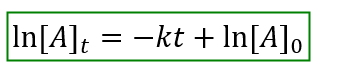# Problem: The decomposition of SO2Cl2 is first order in SO2Cl2 and has a rate constant of 1.44×10−4 s-1 at a certain temperature. If the initial concentration of SO2Cl2 is 1.00 M, how long will it take for the concentration to decrease to 0.78 M ?

###### FREE Expert Solution

The Integrated Rate Laws relates the variable of time to the Rate Law equation.

For a First Order reaction, the integrated rate law is:89% (362 ratings)###### Problem Details

The decomposition of SO2Cl2 is first order in SO2Cl2 and has a rate constant of 1.44×10−4 s-1 at a certain temperature. If the initial concentration of SO2Cl2 is 1.00 M, how long will it take for the concentration to decrease to 0.78 M ?